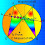## Monday, November 28, 2016

### Geometry Problem 1290 Triangle, Internal Angle Bisector, Median, Parallel, Measurement, Metric Relations

Geometry Problem. Post your solution in the comment box below.
Level: Mathematics Education, High School, Honors Geometry, College.

Click the figure below to view more details of problem 1290.1.https://goo.gl/photos/SxYFtYyAsxydH45r7
Let ME extended meet BC at F
Draw AN //ME . N is on BC extended ( see Sketch)
Observe that ∠BEF=∠BFE= u => triangle EBF is isosceles
∠BAN=∠ANB=u => triangle ABN is isoceles
Since M is the midpoint of AC => F is the midpoint of NC=> FN=FC
AB=BN=FN+FB=FC+x
BC=FC-FB =FC-x
So AB-BC= FC+x-(FC-x)=2x= 5 => x= 2.5

2.With the usual notation for triangle ABC,

DC = ab/(a+c) and so
MD = b/2 - ab/(a+c) = (b/2)(c-a)/(c+a)

Now ME//BD so
(c-x)/x = (b/2)/MD from which
c/x = 1 + (b/2)/MD
= 1 + (c+a)/(c-a) = 2c/(c-a)

Hence x = (c-a)/2 = 5/2 = 2.5

Sumith Peiris
Moratuwa
Sri Lanka

3.Extend AB to point F, such that BF=BC
Angle AFC= Angle ABC/2, hence FC is parallel to ME.
Since M is midpoint of AC,E must be midpoint of AF, AE=(AB+BC)/2. BE=AB-AE=(AB-BC)/2
BE=5/2

4.Problem 1290
Draw AP //BD//ME . P is on AB extended.Is <BCP=<DBC=<DBA=<BPC, then
BP=BC and AM=BC so AE=EP or AB-BE=BD+BE or AB-BC=2.BE or x=2.5.
MANOLOUDIS APOSTOLIS4 HIGH SCHOOL OF KORYDALLOS PIRAEUS-GREECE

5.Extend ME to cut BC at F, construct the parallelogram CFEG; due to AM=CM, ME passes through the midpoint of AG and, as it is the angle bisector of <AEG, tr. AEG is isosceles and AE=GE(=CF). With BF=BE, we are easily done.

6.Let AB = c, BC = a and AC = b
we have DC = ab/a+c , AD = bc/a+c and AM = b/2
Since triangles AME and ADB are similar
=> c-x/(b/2) = c/(bc/a+c)
=> 2c-2x = a+c
=> x = c-a/2 = 2.5

7.Draw CF Perpendic to BD, MN //CF
=> MN = 1/2 AE = 2.5 (MG middle line of AFC)
=> MG=EB (MGBE parallelogram)

1.To c.t.e.o
Where are points F and G?
MN is not necessary, use MG. Thanks

8.G point CF meet BD, F on AB (Tr BFC isoceles)
MN is also middle line of AFC, a way to prove equality of tr MNE and DCB
Thanks